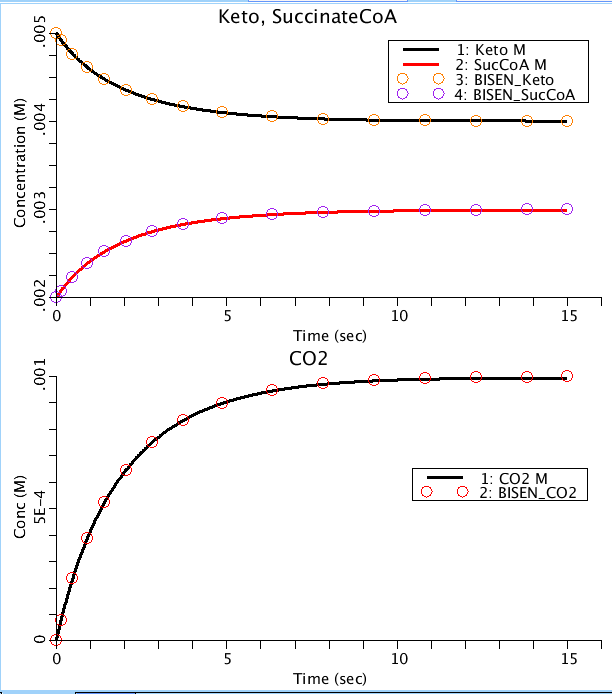# AlphaKetogluterateDehydrogenase_BISEN

Model number
0415

Modeling the Alpha-Ketogluterate Dehydrogenase reaction. Translated from BISEN generated Matlab model.

## Description

``` alpha-Ketogluterate Dehydrogenase (EC 1.2.4.2/EC 2.3.1.61/EC 1.8.1.4):

alpha-Ketogluterate + COAS + NAD + H2O <-> CO2 + SuccinylcoA + NADH + H+

The fourth reaction in the TCA cycle, final point for CO2 release, and is a
multi-enzyme complex much like PDH.

Model generated from The Biochemical Simulation Environment (BISEN).
```Figure 1: Progress curves of the substrates. Circles are output from Matlab version.

## Equations

The equations for this model may be viewed by running the JSim model applet and clicking on the Source tab at the bottom left of JSim's Run Time graphical user interface. The equations are written in JSim's Mathematical Modeling Language (MML). See the Introduction to MML and the MML Reference Manual. Additional documentation for MML can be found by using the search option at the Physiome home page.

References
Key terms
TCA
enzyme
BISEN
Cardiac grid
proton
inorganic phosphate
hydrolysis
magnesium
potassium
Gibbs free energy
thermodynamics
Matlab
SuccinateCoA
Ketogluterate